# SAT Math Multiple Choice Question 653: Answer and Explanation

### Test Information

Question: 653

8. If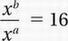and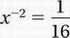, what is the value of b - a?

• A. -2
• B. 2
• C. 4
• D. 8

Explanation:

B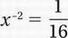Substitute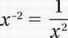(Law #3 from Chapter 9, Lesson 9):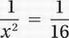Cross-multiply:

x2 = 16

Take the square root:

x = ±4

Recall the other equation: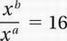Law #6 from Chapter 9, Lesson 9:

xb-a = 16

Substitute x = ±4:

(±4)b-a = 16

Notice that both 42 = 16 and

(-4)2 = 16, therefore:

b - a = 2